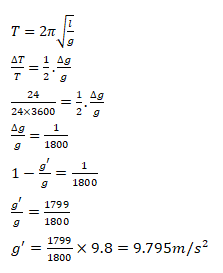# A pendulum clock giving correct time at a place

Question:

A pendulum clock giving correct time at a place where $g=9.800 \mathrm{~m} / \mathrm{s}^{2}$ is taken to another place where it loses 24 seconds during 24 hours. Find the value of $g$ at this new place.

Solution: